Add One Zero Digit in the Front of Number in Microsoft Excel

To change the cell formatting we have 2 options: first is Excel Format Cells option and second is Excel Text formula.

Excel Text function is used to convert a numeric value into Text format by using special format strings. You can combine numbers with text or symbols to display the data in a more readable format.

Syntax of Text function: – = Text (value, format text)
For Example: – cell A1 contains the number 1234
=TEXT (A1,”00000″)
The function will return “01234”.

This function is formatting the cell A1 using a text format and ensuring that the number in the cell has 5 digits in it by putting 5 zeros in the brackets.

Let’s take an example and understand how to put a zero (0)  in front of a number.

We have a list of STD numbers in column A, now we want to add a 0 in front of each number in this list.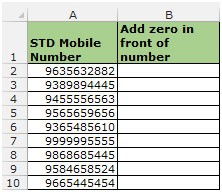To add the zero (0) in front of every number followthe below mentioned steps:

• Select the cell B2, and write the formula.
• =TEXT(A2,”00000000000″)
• Press Enter on the keyboard.
• The function will return the mobile number with the added zero in front of the number.
• Now copy the formula by pressing the key “CTRL+C” on the keyboard and paste in the range B3:B10 by pressing the key “CTRL+V”.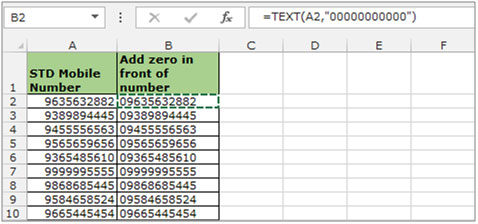Add one zero in front of a number through “Format Cells”

To add the zero (0) in front of every number using the “Format Cells” option follow the below mentioned steps:

• Select the range of mobile numbers.
• And press the key “CTRL+1” on the keyboard.
• The “Format Cells” dialog box will appear..
• In the “Number” tab select “Custom” from the list.
• In the “Type” box write 0 eleven times.
• Click on ok.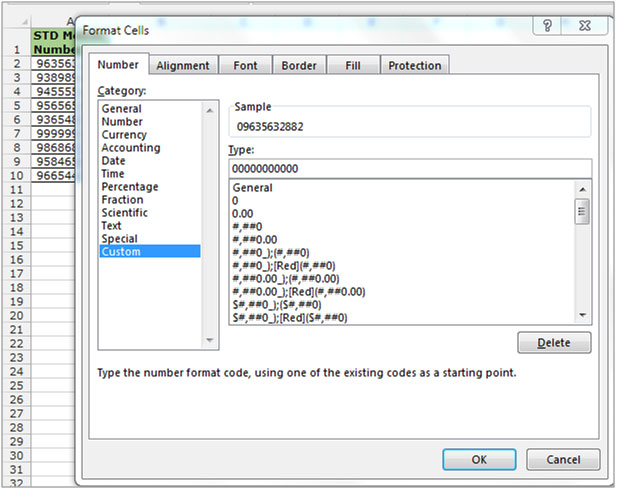You can see that the cell has been formatted directly with a 0 in front of every number. These changes were done in column A itself. In the prior method where we added the 0 through formula, the changes were done in column B.

This formatting ensures that the data is entered in the right format in all the required cells / columns.

If you are working on importing Excel files on a daily basis & you want to prefix zero in front of the all the numbers but not text, then this article is for you. In this article, we will learn how to add zero in front of the number only.

Question): I have a column that contains text as well as numbers. What I want is a formula to update all the numbers present in the column with a zero in front of the number.

Following is the snapshot of data we have: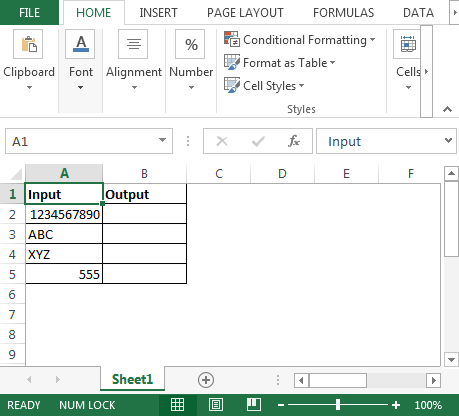• To get the result, we will use a combination of IF & ISNUMBER
• In cell B2 the formula is
• =IF(ISNUMBER(A2)=TRUE,”0″&A2,A2)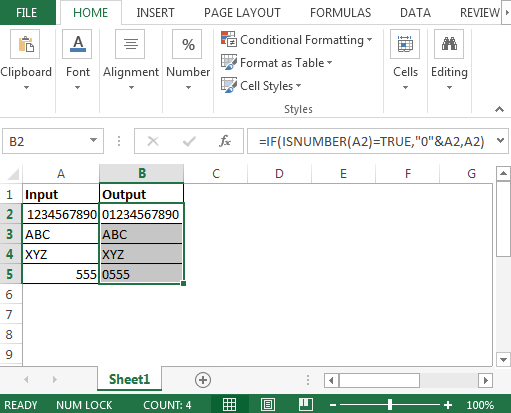In this way, we can add zero number in front of the column to numbers only.Download-Sample File-xlsx

## Users are saying about us...

1.thanx for the quick solutionyou saved mah a lot of time

2.thanx so much..you have made my work easier.

3.I want to use the zero in front of any number only in some cells, those are account numbers. Since the accounts belong to different banks so they all needn’t zero in front of them.

This means What I type that should remain in that format only, like Word. But while I use TEXT format in Excel, it converts them in SCIENTIFIC format, Then I’ve to click each of them to correct formatting of each such cell.

Isn’t that possible to make a formula to convert the numbers (in the pasted sheets of ‘Word’ into ‘Excel’) starting with a special number must have zero & remains not?

Terms and Conditions of use

The applications/code on this site are distributed as is and without warranties or liability. In no event shall the owner of the copyrights, or the authors of the applications/code be liable for any loss of profit, any problems or any damage resulting from the use or evaluation of the applications/code.